# Math in Focus Grade 3 Chapter 4 Practice 4 Answer Key Subtraction Across Zeros

Go through the Math in Focus Grade 3 Workbook Answer Key Chapter 4 Practice 4 Subtraction Across Zeros to finish your assignments.

## Math in Focus Grade 3 Chapter 4 Practice 4 Answer Key Subtraction Across Zeros

Subtract. Then solve.

ExampleQuestion 1.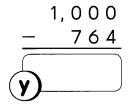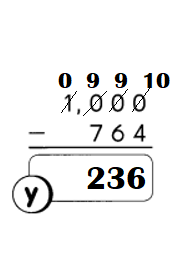Explanation:
1000 – 764 = 236.

Question 2.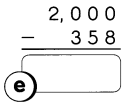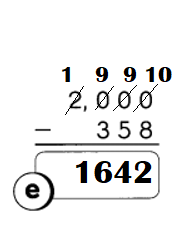Explanation:
2000 – 358 = 1642.

Question 3.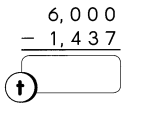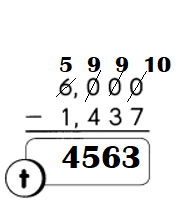Explanation:
6000 – 1437 = 4563.

Question 4.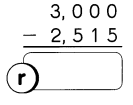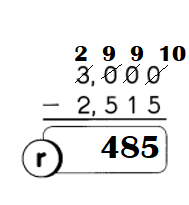Explanation:
3000 – 2515 = 485.

Question 5.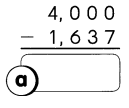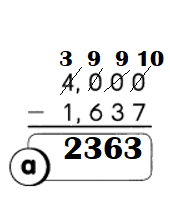Explanation:
4000 – 1637 = 2363.

Question 6.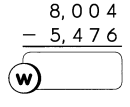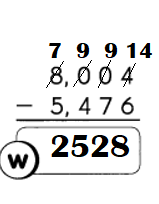Explanation:
8004 – 5476 = 2528.

Question 7.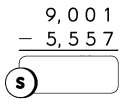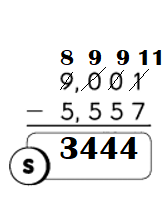Explanation:
9001 – 5557 = 3444.

Question 8.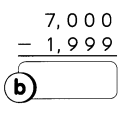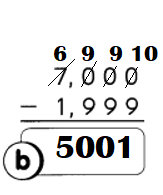Explanation:
7000 – 1999 = 5001.

Question 9.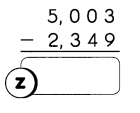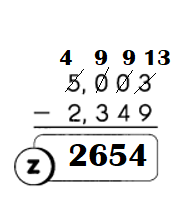Explanation:
5003 – 2349 = 2654.

Write the corresponding letters to find the flavor of the jam.
Question 10.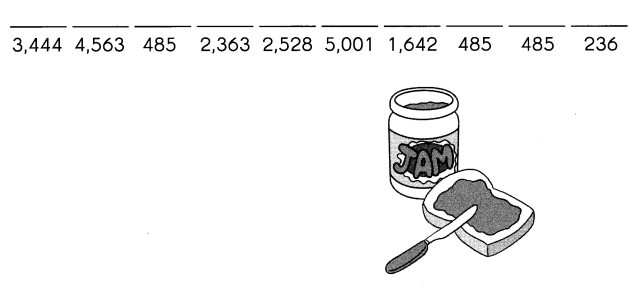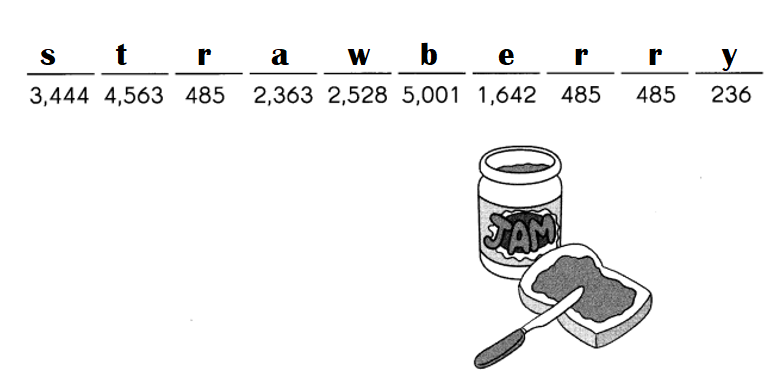Explanation:
1000 – 764 = 236 – y.
6000 – 1437 = 4563 – t.
3000 – 2515 = 485 – r.
4000 – 1637 = 2363 – a.
8004 – 5476 = 2528 – w.
9001 – 5557 = 3444 – s.
2000 – 258 = 1642 – e.
7000 – 1999 = 5001 – b.
5000 – 2349 = 2654 – z.

Example
Sarah has 1.000 stickers.
Maria has 450 stickers.
How many more stickers does Sarah have than Maria?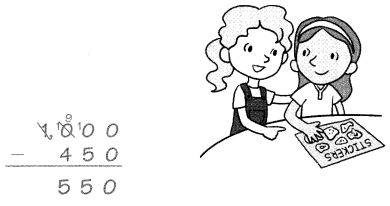Sarah has ___________ more stickers than Maria.

Question 11.
Pete wants to deliver 4,002 oranges to a retirement home. He has 2,157 oranges. How many more oranges does he need?
Pete needs ___ more oranges.